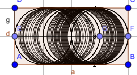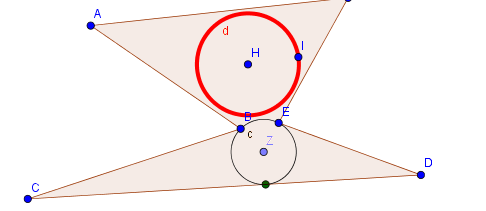# How to calculate the largest circle contained in a polygon?

Erel Segal-Halevi shared this question 5 years ago

The Incircle command returns the largest circle contained in a triangle.

Is there a way to calculate the largest circle contained in a general polygon?I think I have advanced to convex polygons

the method is described in my first post

https://www.geogebra.org/m/uKWRyFw41

Not all polygons can have an inscribed circle.

If such a circle exists, its center (incenter) is the intersection point of all the interior angle bisectors.

So use the GeoGebra tools to check whether such a circle exists, and create its center.1

If the polygon is a quadrilateral, you can check if such a circle exists by adding the length of two opposite sides: if the two sums are equal, an incircle exists.2

Not all polygons can have an inscribed circle but there is ever an internal circle with maxima area. this circle is not unique: a rectangle has infinite circles with maxima areaso I think your request is not possible

saludos1

Simona, I am not looking for an inscribed circle... I am looking for a largest circle.

mathmagic, you are right that largest circle is not unique. So, is it possible to find at least one of the largest circles?1

Sorry, lost in translation :( I've read Incircle, so I thought about inscribed circle1

hello

you can try this: create all triangles with sides over sides of polygon. then create the inscribed circle for each triangle. select the circle with minimum radius (perhaps you must test if the circle is inside the polygon)

I think that the maximum circle has three points or more in the polygon so it is an inscribed circle in some triangle created with sides of polygon

PD: I have tryed it and it does not work well in all cases. I will continue thinking1

Only as draft-idea for an solution with approximation

Klick animation to ON1

The animation looks very nice. Can you explain how this works?1

marvelous work

better we use convex polygons1

I think I have advanced to convex polygons

saludos1

I think I have advanced to convex polygons

the method is described in my first post

https://www.geogebra.org/m/uKWRyFw41

I just found a similar question in StackOverflow, for the special case of a convex polygon:

I have to read and understand it.1

I did this

it is slow because the lists are long. I reduced the lists as much as possible.

it works fine in desktop

saludos1

Fantastico... :)